The Behavoir of Heat Capacity at Low and High Temperatures

Kipp A. Rogers March 23, 2007

(Submitted as coursework for AP272, Stanford University, Winter 2007)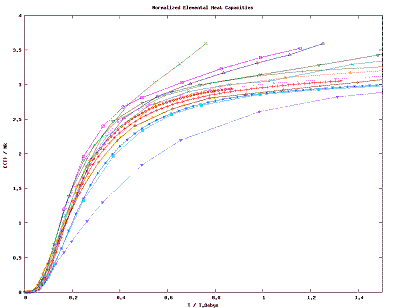Fig. 1: Dimensionless Behavoir of Elemental Specific Heats with Temperature. [3-5]

The Heat Capacity of a solid, C(T) = ∂E/∂T, generally exhibits predictable behavoir in the low and high temperature regimes. In the low temperature limit, barring any phase transitions and magnetic phenomena, the heat capacity exhibits a third order dependence on temperature as well as, for metals, a linear dependence.

Oscillating Modes' Contribution

The cubic dependence can be found  by evaluating the partition function for a Harmonic Oscillator (a crystalline solid is assumed to have its constituents near potential minima):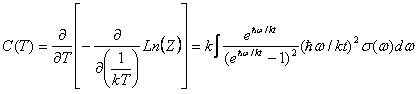where σ(ω) is simply the oscillator density distribution. The Debye Approximation approximates σ(ω) as if the normal modes of the solid were composed of particles free to travel within the solid at the speed of sound, cs. This, along with the requirement that the number of oscillators is triple the number of molecules, gives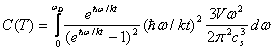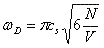This gives an approximate description of the effect of isotropic, low-frequency oscillations on the Heat Capacity. At low temperature the upper limit of the integral becomes infinite yielding the simple form

Material A (mJ/mol/K2) [3,4] TD (K) [2,3,5,7]
Ag 0.65 226
Au 0.729 162.5
Cr 1.59 606
Cs 3.38 40.5
Cu 0.695 344.5
Lu 11.3 183
Mg 1.28 405
W 1.12 383
Y 10.2 256
Zr 2.93 291
Al2O3 0 1035
Ar 0 92
Ge 0 374
Kr 0 71.9
Se 0 152.5
Si 0 645
Table 1: Values of A and TD for materials of interest.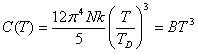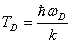We have thus recovered the source of the cubic dependence of the heat capacity at low temperature, lattice vibrations.

Electronic Contribution

For metals, we use the free-electron model to compute the energy: E = ∫ ε ρ(ε) dε, where ρ is the typical electron density function. This gives, in the low temperature limit ,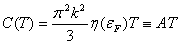where η(εF) is just the density of states at the Fermi Energy. Values of A and TD for relatively well-behaved materials are given in Table 1. We define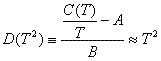for low Temperatures. In this limit, D(T2) should be a straight line of slope 1, giving us a quick test [2-7] of the above relations.

It should be noted that the metals selected were hand-picked for their behavoir in the low temperature regime. The majority of elemental metals behave in less predictable ways at low temperatures; many undergo some sort of superconducting or magnetic phase change. Most of those that do behave nicely are, in fact, of similar structures (the best behaving are Cu, Ag, and Au). Interestingly enough, these have the lowest values of A of any of the sampled metals, implying that perhaps the electrons experience less scattering (free electrons should have A~2/3) . Magnetic interactions are likely candidates for explaining those metals that are less well predicted by the model. They all have more than 1 free electron per atom, and in many cases there will be some magnetic ordering (particularly in the case of something like Cr).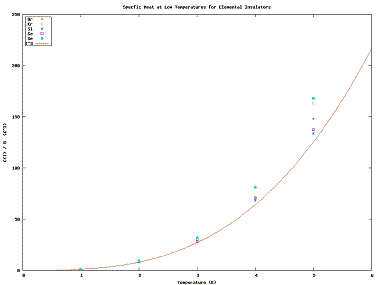Fig. 2: C(T)/B at Low Temperatures for Cubic Insulators. [2,4-6]

High Temperatures and Equipartition

We know from the Equipartition Theorem that the mean energy is given by kT/2 per square term in the Hamiltonian. At high temperatures the non-square terms in the potential become insignificant for a normal solid. This leave only square terms; there are three such kinetic terms and three harmonic oscillator terms in most cases, giving C=3k. We observe this almost universally in Fig. 1; the exceptions are for the most part approaching a phase change.

Alumina [1,2], while it exhibits excellent cubic behavoir at low temperatures, rises to a very high heat capacity even at relatively low temperatures. This means that alumina likely is well-modelled by an oscillator Hamiltonian, except it has several more (30-32) degrees of freedom than the normal 6.

The Data used can be downloaded here. Plots were built using gnuplot.

© 2007 Kipp A. Rogers. The author grants permission to copy, distribute and display this work in unaltered form, with attribution to the author, for noncommercial purposes only. All other rights, including commercial rights, are reserved to the author.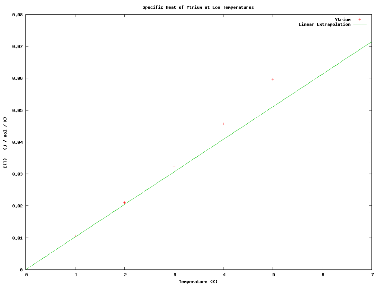Fig. 3: Heat Capacity of Ytrium and Estimated Values from Given value of A at Low Temperature .

References

 Archer, D. G., "Thermodynamic Properties of Synthetic Sapphire (alpha-Al2O3), Standard Reference Material 720 and the Effect of Temperature Scale Differences on Thermodynamic Properties," Chemical Kinetics and Thermodynamics Division (National Institute of Standards and Technology, Gaithersburg, MD, 1992).

 Barron, T. K., White, G. K., Heat Capacity and Thermal Expansion at Low Temperatures, (Kluwer/Plenum, 1999).

 Furukawa, G. T., Saba, W. G., Reilly, M. L., "Critical Analysis of the Heat-Capacity Data of the Literature and Evaluation of Thermodynamic Properties of Copper, Silver, and Gold from 0 to 300 K," U.S. Dept. of Commerce, National Bureau of Standards (U.S. Government Printing Office, Washington, D.C., 1968).

 Hultgren, R. R, Selected Values of the Thermodynamic Properties of the Elements (American Society for Metals, 1973).

 Kagaya, H. M., Soma, T. (updated by M.R. Brozel), "Specific heats of c-Si and molten Si," Properties of Crystalline Silicon. INSPEC (The Institute of Electrical Engineers, 1999).

 Reif, F. Fundamentals of Statistical and Thermal Physics (Mcgraw-Hill, 1965).

 Tari, A., The Specific Heat of Matter at Low Temperatures (Imperial College Press, 2003).